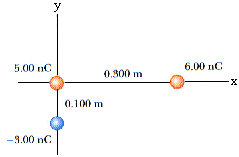# Three charges are arranged as shown in Figure. Find the magnitude and direction of the electrostatic force on the charge at the origin.necessaryh 2021-03-23 Answered
Three charges are arranged as shown in Figure. Find the magnitude and direction of the electrostatic force on the charge at the origin.You can still ask an expert for help

• Questions are typically answered in as fast as 30 minutes

Solve your problem for the price of one coffee

• Math expert for every subject
• Pay only if we can solve itMayme
Force between two charges on x-axis is repulsion force, so the force on charge at origin acts in negative x-direction
${F}_{1}=k\left(6\cdot {10}^{-9}\right)\frac{5\cdot {10}^{-9}}{{\left(0.03\right)}^{2}}=8.98\cdot {10}^{9}\cdot \left(6\cdot {10}^{-9}\right)\frac{5\cdot {10}^{-9}}{{\left(0.03\right)}^{2}}$
$=2993.33\cdot {10}^{-9}$ N
Force between two charges on y-axis is attractive force, so the force on charge at origin acts in negative y-direction
${F}_{2}=k\left(3\cdot {10}^{-9}\right)\frac{5\cdot {10}^{-9}}{{\left(0.01\right)}^{2}}=8.98\cdot {10}^{9}\cdot \left(3\cdot {10}^{-9}\right)\frac{5\cdot {10}^{-9}}{{\left(0.01\right)}^{2}}$
angle made by the net force with positive x-axis = 180 + taninverse $\frac{13470\cdot {10}^{-9}}{2993.33\cdot {10}^{-9}}=180+77.47=257.77$ degrees
magnitude of force $=\sqrt{{13470}^{2}+{2993.33}^{2}}\cdot {10}^{-9}=13798.5\cdot {10}^{-9}$ N